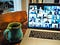Photo by Chris Montgomery on Unsplash

# Conference keying — the Burmester-Desmedt method

With conference keying, we have t participants, and each of these generates a secret value (r_i), and then transmit a public value generated from this (Z_i). Each of the participants then uses these values, and their secret value, and will generate the same secret key (K_i). In the following, we will use the Burmester-Desmedt method , and have five participants, and with varying sizes of a shared prime number (p), and for a common generator (g):

In the Burmester-Desmedt conference keying method, we have t users and then need to generate a common key (K):

First, everyone agrees on a prime number (p) and a generator (g). Next, for each participant i, each user generates a random number (r_i), and then compute a public value:

Each user then shares this value with the rest of the participants. Each participant then computes:

This is equivalent to:

Finally, each participant will compute the same key as:

In the end, the key should be:

The coding is here:

`import randomimport sysfrom Crypto.Util.number import getPrimefrom Crypto.Random import get_random_bytesp=997g=3t=5primebits=64msg="hello"if (len(sys.argv)>1):  primebits=int(sys.argv)if primebits>512: primebits=512p = getPrime(primebits, randfunc=get_random_bytes)z=*tX=*tr=*tK=*tfor i in range(0,t):  r[i] = random.randint(0,p)  z[i]=pow(g,r[i],p)  for i in range (0,t):  X[i]=pow(g,r[(i+1) % t]*r[i]-r[i]*r[(i-1) % t],p)  for i in range(0,t):  K[i] = pow(z[(i-1) % t],(t)*r[i],p)  for j in range(i+1,i+1+t):    if (i==j): continue    K[i]= (K[i]* pow(X[(j-1)%t],(t-j),p))% p  print ("Keys:",K)res=r*r+r*r+r*r+r*r+r*rprint ("Computing keys check: ",pow(g,res,p))`

A sample run is:

`Random values (r): [226589242101802, 189829639746726, 188625714155186, 184735699686400, 116440993319309]Public values (z): [267704455905509, 50015333919247, 15916535078749, 264135697727188, 206738438540103]X values: [112085216125079, 87590296821257, 260726071053053, 50830423926144, 23766863138958]Keys: [155751430205915, 155751430205915, 155751430205915, 155751430205915, 155751430205915]Computing keys check:  155751430205915`

The code is:

## Conclusions

Within conference keying, Eve sits and listens to Alice, Bob, Carol, Dave and Faith, and their broadcast values, but she cannot determine the shared key they will use for their secret communications.

## References

 Burmester, M., & Desmedt, Y. (1994, May). A secure and efficient conference key distribution system. In Workshop on the Theory and Application of of Cryptographic Techniques (pp. 275–286). Springer, Berlin, Heidelberg [here].

Professor of Cryptography. Serial innovator. Believer in fairness, justice & freedom. EU Citizen. Auld Reekie native. Old World Breaker. New World Creator.

## More from Prof Bill Buchanan OBE

Professor of Cryptography. Serial innovator. Believer in fairness, justice & freedom. EU Citizen. Auld Reekie native. Old World Breaker. New World Creator.